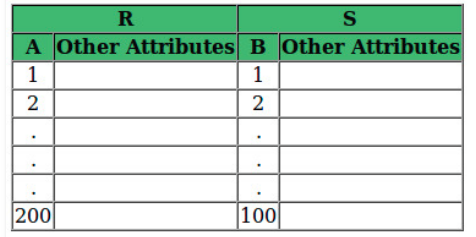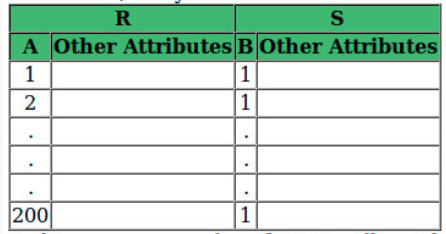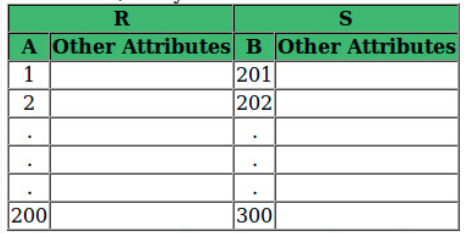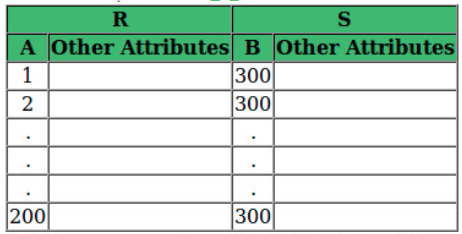How to solve Relational Algebra problems for GATE

• Difficulty Level : Easy
• Last Updated : 23 Jun, 2021

Type-1: Given a relational algebra expression, find the result.
Suppose you have a relation Order(Prod_Id, Agent_Id, Order_Month) and you have to find out what will the following algebra expression return.

Order1.Prod_Id (ρ(Order1,Order) Order1.Prod_Id=Order2.Prod_Id
and Order1.Agent_Id≠Order2.Agent_Id
and Order1.Order_Month=Order2.Order_Month ρ(Order2,Order))

Process the expression starting from innermost brackets.

In this example, we have renamed order to Order1 and Order2 (Both represent the same relation order). Then we have applied the conditional join between Order1 and Order2. It will return those rows where Product_Id and Order_Month of Order1 and Order2 are the same but Agent_Id of Order1 and Order2 is different. It implies the rows where the same product is ordered by two different agents in the same month. Then we are projecting the Prod_Id.

So the final output will return the Prod_Id of products that are ordered by different agents in the same month. We can do this by taking sample data. Let Order relation consists of the following data.

Table – Order

When we apply the following expression, the rows which are highlighted in blue will be selected.

(ρ(Order1,Order)Order1.Prod_Id=Order2.Prod_Id
and Order1.Agent_Id≠Order2.Agent_Id
and Order1.Order_Month=Order2.Order_Month ρ(Order2,Order))

After projecting Order1.Prod_Id, the output will be P002 which is Prod_Id of products that are ordered by at least two different agents in the same month.

Note – If we want to find Prod_Id which are ordered by at least three different agents in same month, it can be done as:

Order1.Prod_IdOrder1.Prod_Id=Order2.Prod_Id
and Order1.Prod_Id=Order3.Prod_Id
and  Order1.Agent_Id≠Order2.Agent_Id
and Order1.Agent_Id≠Order3.Agent_Id
and Order2.Agent_Id≠Order3.Agent_Id
and Order1.Order_Month=Order2.Order_Month
and Order1.Order_Month=Order3.Order_Month(ρ(Order1,Order)X ρ(Order2,Order)X ρ(Order3,Order)))

Type-2: Given two relations, what will be the maximum and minimum number of tuples after natural join?

Consider the following relation R(A,B,C) and S(B,D,E) with underlined primary key. The relation R contains 200 tuples and the relation S contains 100 tuples. What is the maximum number of tuples possible in the natural Join R and S?

To solve this type of question, first, we will see on which attribute natural join will take place. Natural join selects those rows which have equal values for common attribute. In this case, the expression would be like:

σR.B=S.B (RX S)

In relation R, attribute B is the primary key. So Relation R will have 200 distinct values of B. On the other hand, Relation S has BD as the primary key. So attribute B can have 100 distinct values or 1 value for all rows.

Case-1: S.B has 100 distinct values and each of these values match to R.BIn this case, every value of B in S will match to a value of B in R. So natural join will have 100 tuples.

Case-2: S.B has 1 values and this values match to R.BIn this case, every value of B in S will match to a value of B in R. So natural join will have 100 tuples.

Case-3: S.B has 100 distinct values and none of these values matches to R.BIn this case, no value of B in S will match to a value of B in R. So natural join will have 0 tuple.

Case-4: S.B has 1 value and it does not match with R.BIn this case, no value of B in S will match to a value of B in R. So natural join will have 0 tuples.

So the maximum number of tuples will be 100 and min will be 0.

Note – If it is explicitly mentioned that S.B is a foreign key to R.B, then Case-3 and Case-4 discussed above are not possible because the value of S.B will be from the values of R.B. So, the minimum and maximum number of tuples in natural join will be 100.

Reference – Fundamentals of Database Systems bu Navathe